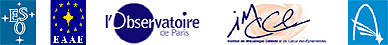Appendix 3

# Parallax of the Sun (the horizontal parallax)

By definition, the parallax of the Sun is the angle β shown below

By trigonometry, tan β = R / r but the angle β is small and tan β can be approximated by β, measured in radians. R is the radius of the Earth and r is the distance from the Earth to the Sun. We can calculate r using the relationship
r = R / β

More details can be found in the EduSheet 2

Back to the EduSheet 3